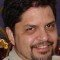Home » Core Java » util » Hashtable » java.util.Hashtable – Hashtable Java ExampleArmando graduated from from Electronics Engineer in the The Public University Of Puebla (BUAP). He also has a Masters degree in Computer Sciences from CINVESTAV. He has been using the Java language for Web Development for over a decade. He has been involved in a large number of projects focused on "ad-hoc" Web Application based on Java EE and Spring Framework.

# java.util.Hashtable – Hashtable Java Example

In this example, we will show the range of functionality provided by the `java.util.Hashtable` hashtable Java class. `Hashtable` was part of the original `java.util` and is a concrete implementation of a `Dictionary`. However, with the advent of collections, `Hashtable` was reengineered to also implement the `Map` interface. Thus, `Hashtable` is now integrated into the Collections Framework. It is similar to `HashMap`, but is synchronized.

Like `HashMap`, `Hashtable` stores key/value pairs in a hash table. However, neither keys nor values can be null. When using a `Hashtable`, you specify an object that is used as a key, and the value that you want linked to that key.

`Hashtable` was made generic by JDK 5. It is declared like this:

`class Hashtable<K, V>`

Here, K specifies the type of keys, and V specifies the type of values.

A hash table can only store objects that override the `hashCode()` and `equals()` methods that are defined by Object. The `hashCode()` method must compute and return the hash code for the object. Of course, `equals()` compares two objects. Fortunately, many of Java’s built-in classes already implement the `hashCode()` method.

Let’s code an example.

## 1. Example of Hashtable Java class

One important point: `Hashtable` does not directly support iterators. Thus, we need to use an enumeration to display the contents of balance.

JavaHashtableExample.java

 01020304050607080910111213141516171819202122232425262728293031323334353637383940 `package` `com.javacodegeeks.examples;` `import` `java.util.Enumeration;``import` `java.util.Hashtable;` `public` `class` `JavaHashtableExample {``    ``public` `static` `void` `main(String[] args) {``        ``// Declare and instantiate the Hashtable.``        ``Hashtable balance = ``new` `Hashtable();` `        ``Enumeration names;``        ``String name;``        ``double` `bal;` `        ``// Insert some keys and values.``        ``balance.put(``"John Doe"``, ``3434.34``);``        ``balance.put(``"Tom Smith"``, ``123.22``);``        ``balance.put(``"Jane Baker"``, ``1378.00``);``        ``balance.put(``"Tod Hall"``, ``99.22``);``        ``balance.put(``"Ralph Smith"``, -``19.08``);` `        ``// Show all balances in Hashtable.``        ``names = balance.keys();` `        ``// Enumerate through the keys of the Hashtable.``        ``while` `(names.hasMoreElements()) {``            ``name = names.nextElement();``            ``System.out.printf(``"%s: %.2f\n"``, name, balance.get(name));``        ``}` `        ``System.out.println();` `        ``// Deposit 1,000 into John Doe's account.``        ``bal = balance.get(``"John Doe"``);``        ``balance.put(``"John Doe"``, bal + ``1000``);` `        ``System.out.printf(``"John Doe's new balance: %.2f"``,``                ``balance.get(``"John Doe"``));``    ``}``}`

Let’s explain the methods used in the above example.

• `public Hashtable()` – Constructs a new, empty hashtable with a default initial capacity (11) and load factor (0.75).
• `public V put(K key, V value)` – Maps the specified key to the specified value in this hashtable. Neither the key nor the value can be null. The value can be retrieved by calling the `get` method with a key that is equal to the original key.
• `public Enumeration keys()` – Returns an enumeration of the keys in this hashtable.
• `public V get(Object key)` – Returns the value to which the specified key is mapped, or null if this map contains no mapping for the key. More formally, if this map contains a mapping from a key k to a value v such that (key.equals(k)), then this method returns v; otherwise it returns null. (There can be at most one such mapping.)

If we run the above code, we will get the following results:

 1234567 `Tod Hall: 99.22``Ralph Smith: -19.08``John Doe: 3434.34``Jane Baker: 1378.00``Tom Smith: 123.22` `John Doe's new balance: 4434.34`

You can obtain set-views of the hash table, which permits the use of iterators. To do so, you simply use one of the collection-view methods defined by `Map`, such as `entrySet()` or `keySet()` as in the following example.

## 2. java.util.Hashtable – Using an Iterator

JavaHashtableIterator.java

 0102030405060708091011121314151617181920212223242526272829303132333435 `package` `com.javacodegeeks.examples;` `import` `java.util.Hashtable;``import` `java.util.Iterator;``import` `java.util.Set;` `public` `class` `JavaHashtableIterator {``    ``public` `static` `void` `main(String[] args) {``        ``// Declare and instantiate the Hashtable.``        ``Hashtable balance = ``new` `Hashtable();` `        ``String name;``        ``double` `bal;` `        ``// Insert some keys and values.``        ``balance.put(``"John Doe"``, ``3434.34``);``        ``balance.put(``"Tom Smith"``, ``123.22``);``        ``balance.put(``"Jane Baker"``, ``1378.00``);``        ``balance.put(``"Tod Hall"``, ``99.22``);``        ``balance.put(``"Ralph Smith"``, -``19.08``);` `        ``// Show all balances in hashtable.``        ``// First, get a set view of the keys.``        ``Set keys = balance.keySet();` `        ``// Get an Iterator.``        ``Iterator iterator = keys.iterator();` `        ``// Iterate.``        ``while` `(iterator.hasNext()) {``            ``name = iterator.next();``            ``System.out.printf(``"%s: %.2f\n"``, name, balance.get(name));``        ``}``    ``}``}`

Let’s explain the methods used in the above example.

• `public Set keySet()` – Returns a `Set` view of the keys contained in this map. The set is backed by the map, so changes to the map are reflected in the set, and vice-versa. If the map is modified while an iteration over the set is in progress (except through the iterator’s own remove operation), the results of the iteration are undefined. The set supports element removal, which removes the corresponding mapping from the map, via the `Iterator.remove`, `Set.remove`, `removeAll`, `retainAll`, and `clear` operations. It does not support the `add` or `addAll` operations.

If we run the above code, we will get the following results:

 12345 `Tod Hall: 99.22``Ralph Smith: -19.08``John Doe: 3434.34``Jane Baker: 1378.00``Tom Smith: 123.22`

You can download the source code of this example from here: java.util.Hashtable – Hashtable Java Example

# Do you want to know how to develop your skillset to become a Java Rockstar?

## Subscribe to our newsletter to start Rocking right now!

### and many more ....